Next: Worked example 10.4: Truck Up: Statics Previous: Worked example 10.2: Rod

## Worked example 10.3: Leaning ladder

Question: A uniform ladder of mass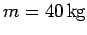and length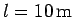is leaned against a smooth vertical wall. A person of mass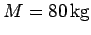stands on the ladder a distance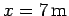from the bottom, as measured along the ladder. The foot of the ladder is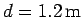from the bottom of the wall. What is the force exerted by the wall on the ladder? What is the normal force exerted by the floor on the ladder?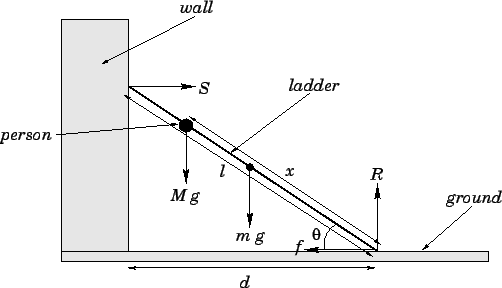Answer: The anglesubtended by the ladder with the ground satisfies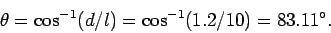Letbe the normal reaction at the wall, letbe the normal reaction at the ground, and letbe the frictional force exerted by the ground on the ladder, as shown in the diagram. Consider the torque acting on the ladder about the point where it meets the ground. Only three forces contribute to this torque: the weight,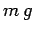, of the ladder, which acts half-way along the ladder; the weight,, of the person, which acts a distancealong the ladder; and the reaction,, at the wall, which acts at the top of the ladder. The lever arms associated with these three forces are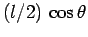,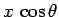, and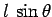, respectively. Note that the reaction force acts to twist the ladder in the opposite sense to the two weights. Hence, setting the net torque to zero, we obtain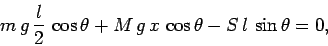which yields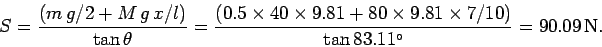The condition that zero net vertical force acts on the ladder yields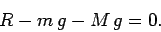Hence,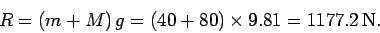Next: Worked example 10.4: Truck Up: Statics Previous: Worked example 10.2: Rod
Richard Fitzpatrick 2006-02-02